﻿ Physico-mathematical Conceptual Difficulties among First Year Students Learning Introductory University Physics

### Physico-mathematical Conceptual Difficulties among First Year Students Learning Introductory Univers...

Kasimba Phebby Mwangala, Overson Shumba

American Journal of Educational Research

## Physico-mathematical Conceptual Difficulties among First Year Students Learning Introductory University Physics

Kasimba Phebby Mwangala1, Overson Shumba2,1Science Department, Highridge Secondary School, Kabwe, Zambia

2School of Mathematics and Natural Sciences, Copperbelt University, Zambia

### Abstract

This descriptive survey at Kwame Nkrumah University in Kabwe, Zambia, explored difficulties that first year students (n= 116) experienced and perceived with physico-mathematical concepts in an introductory physics course. The data on difficulties was collected using a physics achievement test and a focus group discussion session while a questionnaire was employed to assess perceptions of difficulties associated with the physico-mathematical topics and concepts in the test. The students’ performance in the test was unsatisfactory where the scores ranged from 16% to 46.9% and averaged 33.0% in the five-question test. They had difficulties in creating and interpreting formulae and data and unsatisfactorily identified, combined and applied physico-mathematical concepts in the test items. The questionnaire survey, more than 60% of the students were likely to rate lecture topics on topics such as speed, work, and energy as not difficult. This number significantly decreased to around 35% when asked to rate the use, application or derivation of specific physico-mathematical concepts in the test. For example, 69.8% rated topic or concept ‘acceleration’ not difficult and 35.4% expressed having to formulate the equation a= (vf-vi)/t using information from a graph in the test as ‘difficult’. The findings of this study suggest that students need close support to comprehend physico-mathematical relations and their interpretation deeply.

• Kasimba Phebby Mwangala, Overson Shumba. Physico-mathematical Conceptual Difficulties among First Year Students Learning Introductory University Physics. American Journal of Educational Research. Vol. 4, No. 17, 2016, pp 1238-1244. http://pubs.sciepub.com/education/4/17/8
• Mwangala, Kasimba Phebby, and Overson Shumba. "Physico-mathematical Conceptual Difficulties among First Year Students Learning Introductory University Physics." American Journal of Educational Research 4.17 (2016): 1238-1244.
• Mwangala, K. P. , & Shumba, O. (2016). Physico-mathematical Conceptual Difficulties among First Year Students Learning Introductory University Physics. American Journal of Educational Research, 4(17), 1238-1244.
• Mwangala, Kasimba Phebby, and Overson Shumba. "Physico-mathematical Conceptual Difficulties among First Year Students Learning Introductory University Physics." American Journal of Educational Research 4, no. 17 (2016): 1238-1244.

 Import into BibTeX Import into EndNote Import into RefMan Import into RefWorks

12
Prev Next

### 1. Introduction

Research suggests that inabilities of students at the secondary school level and in undergraduate university courses to identify, combine and apply physics and mathematical concepts and laws to solve physics problems in a variety of situations may account for unsatisfactory achievement in physics courses . It has been posited that the problem may be structural where students are not able to translate between physics and mathematicsconcepts rather than fail to work with numbers . For example in physics, equations are seen as relations of entities, relationships between the results of measurements and not as calculation schemes like in mathematics . Blending physical meaning with mathematical symbols generally affects interpretation of physical quantities .

It may be the difficulties in comprehending physico-mathematical concepts that may account for the reported high failure rates in secondary school and university physics across the globe [1, 4] and across African countries, e.g., South Africa [5, 6], Nigeria , Kenya , and Zambia . In Zambia, the Examinations Council  reported that only 48.33% passed the O-level physics examinations. Studies have shown that failure to understand and apply the relationship between physics and mathematics concepts to solve physics problems and the difficulties with identification and application of physics and mathematical concepts and laws to solve physics problems in a variety of situations may account for unsatisfactory levels of achievement [1, 2, 3, 11, 12]. The lack of understanding of physico-mathematical concepts may be the underlying cause. Physico-mathematical concepts are concepts that involve the use of both physics and mathematics principles to define, derive or solve a physics or mathematics problem [3, 13]. They require one to internalize the physical aspects and to express these physical aspects mathematically,

### 2. Purpose of the Study

The aim of this study was to identify and analyse physico-mathematical conceptual difficulties among first year students after they had completed an introductory university physics in Zambia. It addresses the two research questions:

i) What are the difficulties associated with specific physico-mathematical concepts among students who had completed first year university physics course PHY100?

ii) What are the students’ perceptions about specific physico-mathematical concepts in a first year university physics course PHY100?

Addressing these questions is important for understanding physico-mathematical conceptual difficulties to learning PHY100. Physico-mathematical concepts involve the structural use of both physics and mathematics principles to define, derive or solve a physics problem, and thus isolating those that are difficult or perceived by students to be difficult is quite pertinent [1, 2, 3, 11, 12]. This is valuable to create a knowledge base for handling students’ difficulties with these concepts. It has also been analysed that, from the structural relationship point of view, the “language” of mathematics in physics does not coincide with the one used in mathematics since the mathematics in physics gives meaning to physical systems rather than expressing abstract relationships [3, 12]. However, physics is more than just a context for the application of mathematics . This study explores the potential implications of this to the wider context of quality physics education.

### 3. Methodology and Research Procedures

A descriptive survey design was used to identify the physico-mathematical conceptual difficulties among students who had completed PHY 100 in the School of Education at the Kwame Nkrumah University in Zambia. This university is Zambia’s largest teacher education university, founded in 1971, originally as a college of education. The study involved 140 students who had completed PHY 100 in 2015 and were in the 2016 first term of the second year of the Bachelor of Education degree. Out of the 140 students 24 opted not to participate in the study leaving the sample size at 116 students representing 83%.

The survey was conducted, first,by administering a researcher developed physics test to gauge achievement, second, by administering a questionnaire on perceptions and, third, by conducting an 8-member focus group discussion to cross-validate understanding and perceptions concerning physico-mathematical concepts. The test was developed by the researcher to investigate the conceptual difficulties using test items modified from the textbook for PHY 100, Physics for scientist and engineers by Serway Jewett (2004, 6th edition). The tests carried five (5) items focusing on physico-mathematical concepts associated with the topics, displacement and speed (question 1), force and motion (question 2), angular velocity (question 3), centripetal force and coplanar forces(question 4), and work and energy (question 5).

The questionnaire consisted of two sections. In the first section, they responded to ten items (10) on the perceived level of difficulty when the topics or concepts such as speed, work, energy, and force were presented to them in the lectures. They expressed perceptions of difficulty by responding on the scale: (1) very difficult, (2) difficult, (3) uncertain, and (4) not difficult. In the second section, they were presented with an example of a physico-mathematical concept or relationship that was in the test and a statement on the physico-mathematical concept to which they could agree or disagree depending on whether the demands were easy or difficult. As an example, they were to rate an item based on the physico-mathematic concept of displacement, ∆x= xf− xi in question 1. This was followed by the statement: ‘Extraction of information from the graph to find the displacement using this equation was easy’. This was to be rated on the scale: (1) strongly disagree, (2) disagree, (3) uncertain, (4) strongly agree, and (5) agree. A focus group discussion  enabled cross-validation of the results.

### 4. Results and Findings

Results of analysing the performance of students on the physics test to gauge achievement were triangulated with results obtained by analysing the test scripts, the focus group discussion, and the questionnaire survey of perceptions. Overall, the results point to several difficulties associated with physico-mathematical concepts and equations.

4.1. Students’ Achievement in the Physico-mathematical Concept Test

Overall, students achieved unsatisfactorily on the test as shown in Figure 1where the average score was only 33.0% and ranging from 16.6% in question 2 to 46.9% in question 1.Students performed best on question 1 (displacement and speed, 46.9%) and question 5(work and energy, 42.6%). The worst performance was associated with question 2 (force and motion, 16.6%) and question 3 (angular velocity, 20%). Overall, the majority of the students failed the questions.Download asVeiw figureFigures index
Figure 1. Students’ performance in the test (N = 116)
4.2. Difficulties with Physico-mathematical Concepts in Students’ Scripts

Table 1 shows the results of analysing the answer scripts and categorising the nature and examples of physico-mathematical conceptual difficulties that were encountered. The difficulties associated with the concepts in the test fell into six categories. The number of students that experienced each difficulty expressed as percentage of the total number of students (116) who participated in the test. More than two thirds of students had difficulties associated with creating a formula on the basis of physico-mathematical reasoning (n = 116; 100%); identifying and interpreting appropriate formulae and physico-mathematical principles (86.2%); extracting physico-mathematical information from diagrams and graphs (78.5%); and applying mathematical principles to the physics problems (69.0%).

#### Table 1. Categories and Examples Of Physico-Mathematical Difficulties Encountered By Students (n = 116).

It is noteworthy that most students have difficulties in physico-mathematical relations in formula. In a focus group discussion, one participant student explained as follows:

Yes, the challenge I faced myself, was the formulation of the formulas, they were like, during the test like he said, ahh I used the theory we learnt in class but when I came up with my answer, on the multiple choice there was nothing. So, I was like yeah… let me just leave it and go to the next question [laughter].And the other challenge is that, when this question came, what came in people’s mind was the simple pendulum, they were not thinking about circular motion yeah. So, that also contributed to….They thought we were about to calculate the…the period [laughter] (Student, focus group discussion)

With respect to the first difficulty (Table 1), all students had difficulties creating the formula for displacement i.e., ∆x= xf− xi and average velocity, i.e., average velocity= ∆x/∆t instead they were using an equation for the position given in question 1, x= 4t+ 2t2. For example on question 1(a) in the test, the students were required to create the formula for finding displacement, ∆x= xf− xi but instead, the majority created incorrect formulae as in Sample 1.Download asVeiw figureFigures index
Sample 1. Students difficulties with formula

Students were also using f=ma, v2=u2+ 2as and v=at to find speed of the block instead of Ws=1/2mv2f -1/2mv2i on question 2 that focused on what happens when a spring that has a force constant of 1.0×103N/m is compressed 2.0cm and is then released from rest.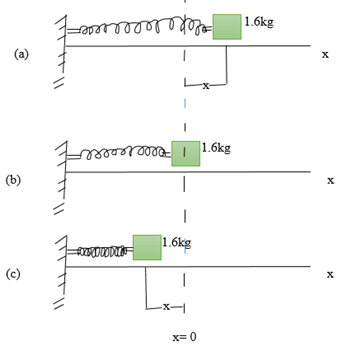With respect to the second category, 78.5% failed to identify and use correct principles to find the sum of forces acting on an object in the y and x directions on question 4. Question 4 involved finding the tensions T1, T2 and T3 in a system in equilibrium where a block of wood weight 250 N hangs from three wires and make the angles θ1= 40.0° and θ2= 30.0° with the horizontal.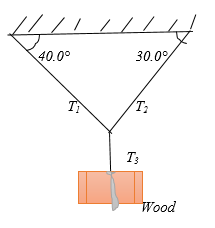For example students were equating –T1sin40°= 250N, –T1cosθ1= –T2cosθ2, T1+ T2= T3, T3=cos30°, and T2=T3. The students also wrongly equated –mg= Tsinθ and –mgsinθ= mv2/r on question 3 that required finding an expression for a revolving suspended object. (ν=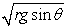; ν= rlgcosθ; ν= rlsinθ; and ν=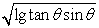). The majority of students did not know the correct interpretation of ∆KE, KE and their relationship to work, i.e., students used ∆KE= W= f×d and 1/2mv2 = f×d. Instead of identifying and resolving the sum of forces into x and y components and then applying the appropriate principles to find T1, T2, and T3 on question 4 most students equated T1sinө2= T2cosө2 and T2cosө1= T2sinө1 as shown in sample 2.Download asVeiw figureFigures index
Sample 2. Students’ difficulties with identifying and interpreting appropriate formulae and physico-mathematical principles

Over two thirds (69%) of students had difficulties in applying mathematical principles based on physico-mathematical reasoning to solve a physics problem. For example, in Sample 3 a student applied both cosө2 and cosө1 as if they were equal to each other. As a result, he/she ended up substituting the wrong value and obtained a wrong answer:Download asVeiw figureFigures index
Sample 3. Students’ difficulties with applying mathematics to solve physics problems

Other categories of difficulties are summarised in Table 1.

4.3. Perceptions Concerning Physico-mathematical Concepts

Student perceptions concerning the difficulties surrounding physico-mathematical concepts and relations expressed in equations they had experienced in the test were evaluated via responses to a section of the questionnaire. They assessed their perceptions of ease or difficulty of ten (10)statements based on physico-mathematical concepts contained in the test on the scale: (1) strongly disagree, (2) disagree, (3) uncertain,(4) strongly agree, and (5) agree. In Table 2 the ‘agree’ and ‘strongly agree’ responses were aggregated to indicate “not difficult” and those pertaining to ‘disagree’ and ‘strongly disagree’ responses were aggregated to indicate “difficult”. The results exclude nine (9) students who did not respond.

The majority of the students perceived derivation or application of four out of the ten physico-mathematical concepts ‘not difficult’. Those not perceived to be difficult related to deriving the expression v2 = lgtanөsinө (68.8%), using the formula Ek=1/2mv2 (60.9%), applying the concept of centripetal force Fc= mv2/r (58.72%), and finding work using the equation W =1/2kx2 (52.29%). However, the students were split in their perceptions on four items. For example, 42.99% found it ‘difficult’ compared to 38.32% who perceived it ‘not difficult’ to formulate the equation a= (vf-vi)/t from the graph. In the formulae ῡx= ∆x/∆t= (xf− xi)/ ∆t, 42.98% compared to 40.35% perceived it difficult to differentiate xf from xi when substituting their respective values.

#### Table 2. Students’ Perceptions of Physico-Mathematical Concept Used In The Test (N = 116)

In another section of the questionnaire, students were presented with the ten physico-mathematical concepts (displacement, speed, acceleration, position, average velocity, work, force, centripetal force, centripetal acceleration, and kinetic energy) and asked to rate their perceived difficulty during lectures in which they were taught. They expressed perceptions of difficulty by responding on the scale: (1) very difficult, (2) difficult, (3) uncertain, and (4) not difficult.

Table 3 summarises the results after aggregating very difficult and difficult responses to give the percent of students who viewed the physico-mathematical topic/concept as “difficult”. This enabled the comparison of the aggregated ratings (perceptions of difficulty in lectures L) against specific concepts and operations they were expected to perform in the test (perceptions of difficulty in test T) as shown in Table 2. This provided a way to gauge and to compare the difference or change in perceptions of difficulty the students held for lectures and the test employed in the study. This resultant change is reflected in the last column of Table 2 i.e., L-T and that may be interpreted as follows. A value of 0% represents no change. For example, on the topic ‘velocity’ the number of student teachers who expressed perceived difficulty on the topic (39.7%) was similar to the number of students who expressed perceived difficulty with the operation to differentiate xf from xi when substituting their respective values in the ῡx= ∆x/∆t= (xf− xi)/∆t in the test.

As can be noted in the Table 3, the change was negative for eight (8) physico-mathematical topics and concepts shown. This negative change was greatest (-58.6%) for the topic speed perceived difficult by only 11.2% of the students in lectures and where 69.8% of the students perceived deriving the expression v2 = lgtanөsinө was expressed as difficult. A change of more than -10% suggests that the students were suggesting that they could rate physics topics as not difficult and yet when presented with use, application or derivation of specific physico-mathematical concepts in the test they perceived and had trouble with. For example, they may not have difficulty with a topic or concept ‘acceleration’ (69.8% rating not difficult) but will express difficulty (35.4%) when having to formulate the equation a= (vf-vi)/t using information from a graph.

### 5. Discussion of the Findings

A high proportion of undergraduate students in the present case study demonstrated as well as perceived difficulties with a range of physico-mathematical concepts and the equations that relate them. This was found in the unsatisfactory performance in the physics test and their representation of the concepts in test scripts and the perceptions expressed in the questionnaire and focus a group discussion. Over 80% of students had difficulty in interpreting formula, a result that has been reported with a sample of 20 American students in a first-semester physics course . The course covered acceleration, velocity, speed, time, force, position, and their numerical, graphical, and functional representations. The students in Nguyen and Rebello did not fully understand the meaning of formulae and equations from both a mathematical and physical point of view. This led to failure to interpret formulas and subsequently to creation of wrong expressions. In the present study, 19.8% of students could not differentiate one concept from another. This difficulty was observed on similar concepts such as centripetal acceleration, centripetal force, force of gravity, work and energy, probably because the students did not understand the connections between these concepts and how they could be differentiated. It is expected that a student who cannot differentiate concepts will find it difficult to identify correct formula and more so interpret them. The reason for assuming that two expressions should be equal or different comes from understanding of physical principles and not from logical reasoning . The reason why students had difficulty with differentiating concepts could be related to the finding from our focus group discussion where students reported that some were not ready for the test even after being given two weeks to revise and prepare for it.

Studies have shown that blending physical meaning with mathematical symbols generally affects interpretation of physical quantities and physical equations [2, 3]. Physics equations are seen as relations of entities, i.e., relationships between the results of measurements and not the results of calculation schemes like in mathematics [2, 3]. The poor performance of students in the test used in thepresent study could be linked to students’ difficulties in applying mathematics to physics problems. For example, students had difficulties with combining trigonometric functions to create expressions or derive equations to solve physics problems. The students’ difficulties with mathematics application to physics concepts to solve the physical problems may not lie in the technical demands of calculating but rather in the structural aspects of translating between physics and mathematics.

In order to support the explanation for students’ difficulties with structural application of mathematics to physics problems, students’ performance in question one (1)was analysed. This question involved numerical values and less symbolic connection between mathematics and physics and it read as follows:

Question 1: A particle moves along the x axis. Its position varies with time according to the expression x= −4t+ 2t2 where x is in meters and t is in seconds. The position –time graph for this motion is shown in figure 1 below not drawn to scale. Note that the particle moves in the negative x direction for the first second of motion, is momentarily at rest at the moment t= 1s, and moves in the positive x direction at times t> 1s.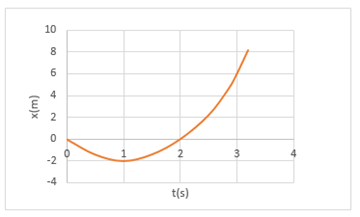i) Determine the displacement of the particle in the time interval t=1s to t= 3s.(a) -2m; (b) 8m; (c) -4m; (d) 4m.

ii) Calculate the average velocity during the time interval t= 1s to t= 3s. (a) 4m/s; (b) -2m/s; (c) 3m/s; (d) 8m/s.

The students performed better on this question than on other questions in the test that required more interpretation of symbols. It has been suggested that questions with many symbols usually contain a large amount of information that is implicitly encoded and context dependent and that undergraduate students may not be able to handle effectively . Besides this, it has been posited that students’ difficulties with mathematics in physics may be related to the failure to know which principles or aspects of mathematics are necessary for solving a particular physics problem .

It becomes an issue in physics education to ensure that students are fully supported to comprehend the physico-mathematical concepts and their relationships in equations. In this study, the terms “show”, “find” and “derive” demanded students to decode information and use physical and mathematical principles and formulae in their problem solving process. This is as exemplified by the following question:

Question 4. A block of wood weight 250 N hangs from three wires as suggested in figure 4 below. Two of the wires make angles θ1= 40.0° and θ2= 30.0° with the horizontal.If the system is in equilibrium, (i) show that the tensions T1= 230.40N, T2= 203.80 and T3= 250N in the wires.Show your working and explain how you arrived at the answer for each tension. (ii) Find the tension in the left-hand wire. Show your working and explain how you arrived at the answer.

a) T1= T2cos θ2 /sinθ2

b) T1= Fgcos θ2 /sinθ1

c) T1= T2cos θ2 /cos(θ1+ θ2)

d) T1= Fg cos θ2 /sin (θ1+ θ2 )

Students did not use correct principles to find sum of forces and the application of sine and cosine rules.

### 6. Conclusion

Overall, this study provides insights into students’ physico-mathematical conceptual difficulties when solving physics problems involving physico-mathematical concepts. Some of examples of difficulties include differentiating one concept from another, identifying and interpreting appropriate formulae and principles, creating a formulae, application of mathematics to the physics problems, using physics and mathematics concepts to create schematic diagrams, and deducting information from diagrams and graphs. These are elaborated in a thesis on which this paper is based . The physico-mathematical difficulties outlined in this study call for a change in which physics is taught to stress deep understanding of physics concepts. Students ought to be supported to develop conceptual understanding of physics concepts and use mathematical representation to show their physico-mathematical relations. Asproposed in previous studies [14, 16], it is important to ascertain that students achieve confidence demonstrate capability for multiple representation and symbols. The structural relationship between physics and mathematics is important . As such, in order to improve students’ ability to interpret formulae and create meaningful expressions, lecturers must spend time explaining the physical meaning of each formula and how it relates the physical concepts being studied. Further to this, there is need for further research to characterise and understand in detail physico-mathematical variables that might influence student performance in secondary and post-secondary school physics.

### References

  Mullis, I.V.S., Martin, M.O., Robitaille, D.F., & Foy, P. (2009). TIMSS Advanced 2008 International Report. Boston: TIMSS & PIRLS International Study Center.In article  Uhden, O. & Pospiech, G. (2011). Mathematics in physics: Analysis of students' difficulties. Retrieved from: www.esera.org/media/ebook/strand3/ebook-esera2011_UHDEN-03.pdf.In article  Vinitsky-Pinsky, L. & Galili, I. (2014). The need to clarify the relationship between physics and mathematics in science curriculum: cultural knowledge as possible framework. Procedia - Social and Behavioral Sciences, 116, 611-616.In article View Article  Nilsen, T., Angell, C. & Gronmo, L.S. (2013). Mathematical competencies and the role of mathematics in physics education: A trend analysis of TIMSS Advanced 1995 and 2008. Acta Didactica Norge, 7(1), Art.6.In article  Mji, A. & Makgato, M. (2006). Factors associated with high school learners' poor performance: a spotlight on mathematics and physical science. South African Journal of Education, 26(2); 253-266.In article  Rollnick, M. (2009). Key challenges in science education: Education in South Africa today. Accessed 15 October 2015 at http://www.sabceducation.co.za.In article  Ajao, I.S. & Awogbemi, A.C. (2012). A correlational analysis of student’ achievement in mathematics in WAEC and NECO mathematics. Journal of Education and Practice, 3(1). Retrieved 6 June 2013 from http://www.slideshare.net/AlexanderDecker/11a-correlational-analysis-of-students-achievement-in-waec-and-neco-mathematics.In article  Konyango, O.B. (2011). Impact of resource utilization on the performance of physics in KCSE in public secondary schools in Ugunja/Ugenya districts, Kenya. Unpublished Master of Education thesis, Kenyatta University, Kenya.In article  Ndashye, M. (2007). Response of administrators to poor performances in O-level Physics examinations: The case of selected high schools in Copperbelt province. Unpublished Master of Education thesis, University of Zambia, Lusaka, Zambia.In article  Examination Council of Zambia. (2014). Examination performance report: Natural Sciences. Ministry of Science and Technology, Lusaka, Zambia.In article  Pepper, R.E., Chasteen, S.V., Pollock, S.J., & Perkins, K. (2012). Observations on student difficulties with mathematics in upper-division electricity and magnetism. Phys. Rev. ST Physics Education Research, 8, 010111.In article View Article  Redish, E.F. (2005). Problem solving and the use of math in physics courses. Proceedings of the Conference ‘World View on Physics Education in 2005: Focusing on Change’, Delhi, India, August 21-26, 2005In article  Uhden, O., Karam, R., Pietrocola, M. (2012). Science and Education, 21, 485.In article View Article  Nguyen, D. & Rebello, N.S. (2011). Students’ Difficulties with Multiple Representations in Introductory Mechanics.US-China Education Review, 8(5), 559-569.In article  Karam, R., Pospiech, G., & Pietrocola, M. (2011). Mathematics in physics lessons: developing structural skills. In GIREP-EPEC & PHEC 2009 International Conference August 17-21, University of Leicester, UK.In article  Torigoe, E. (2012). How numbers help students solve physics problems. At http://arxiv.org/pdf/1112.3229.pdf.In article  Krueger, R.A. (1988). Focus groups: A practical guide for applied research. Newbury Park, CA: Sage publications.In article  Mwangala, P.K. (2016). Physico-mathematical conceptual difficulties among first year students learning introductory physics PHY 100 at Kwame Nkrumah University. Unpublished Masters of Science in Physics Education thesis, Copperbelt University, Zambia.In article